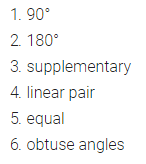# Fill in the blanks: If two angles are complementary, then the sum of their measures is

Fill in the blanks:

1. If two angles are complementary, then the sum of their measures is
2. If two angles are supplementary, then the sum of their measures is
3. Two angles forming a linear pair are
4. If two adjacent angles are supplementary, they form a
5. If two lines intersect at a point, then the vertically opposite angles are always
6. If two lines intersect at a point, and if one pair of vertically opposite angles are acute angles, then the other pair of vertically opposite angles are